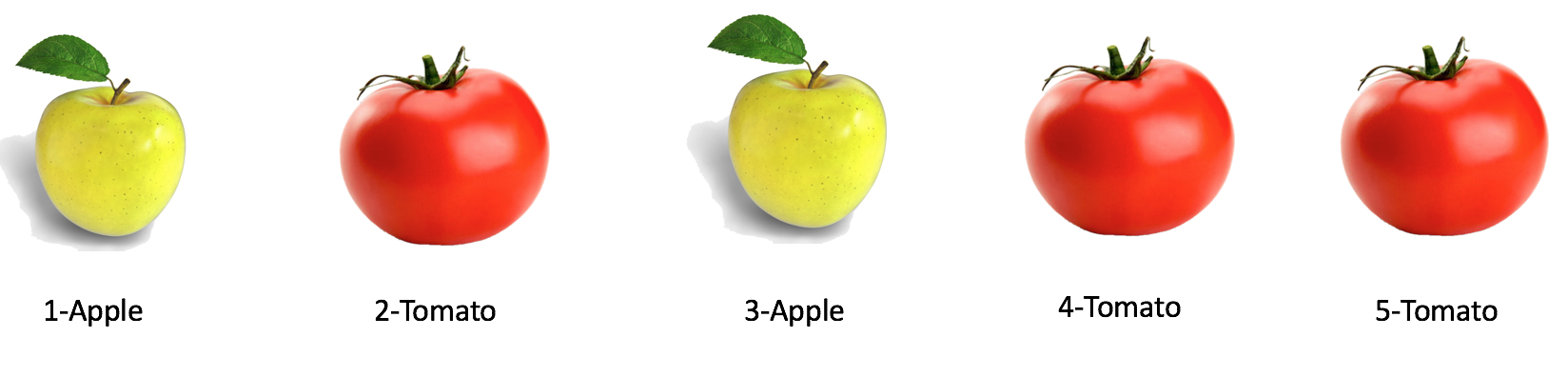In this post, I will show you how to use a bootstrap aggregating classification algorithm (do not leave yet, I will explain it with apples and tomatoes!). This algorithm is implemented in the new version of nnetsauce (v0.2.0) and is called `randomBag`. The complete list of changes included in this new version can be found here.

nnetsauce (v0.2.0) can be installed (using the command line) from Pypi as:

``````pip install nnetsauce
``````

The development/cutting-edge version can still be installed from Github, by using:

``````pip install git+https://github.com/thierrymoudiki/nnetsauce.git
``````

Let’s start with an introduction on how `randomBag` works, with apple and tomatoes. Like we’ve seen for Adaboost, `randomBag` is an ensemble learning method. It combines multiple individual statistical/machine learning (ML) models into one, with the hope of achieving a greater performance. We consider the following dataset, containing 2 apples, 3 tomatoes, and a few characteristics describing them: shape, color, size of the leaves.3 individual ML models are tasked to classify these fruits (is tomato… a fruit?). That is, to say: “given that the shape is x and the size of leaves is y, observation 1 is an apple”. For all the 5 fruits. In the `randomBag`, these individual ML models are quasi-randomized networks, and they typically classify fruits by choosing a part of each fruit’s characteristics, and a part of these 5 fruits with repetition allowed. For example:

-ML model #1 uses shape and size of the leaves, and fruits 1, 2, 3, 4

-ML model #2 uses shape and color (yes, here apples are always green!), and fruits 1, 3, 3, 4

-ML model #3 uses color and size of the leaves, and fruits 1, 2, 3, 5

Then, each individual trained model provides probabilities to say, for each fruit, “it’s an apple” (or not):

Fruit# ML model #1 ML model #2 ML model #3 is an apple?
1 79.9% 48.5% 35.0% yes
2 51.5% 11.1% 20.0% no
3 26.1% 5.8% 90.0% yes
4 85.5% 70.5% 51.0% no
5 22.5% 61.3% 55.0% no

How to read this table? Model #1 estimates that fruit #2 has 51% of chances of being an apple (thus, 49% of being a tomato). Similarly, model #3 estimates that, given its characteristics, fruit #5 has 55% of chances of being an apple. When a probability in the previous table is > 50%, the model decides “it’s an apple”. Therefore, here are ML models’ classification accuracies:

Accuracy ML model #1 ML model #2 ML model #3
40.0% 20.0% 40.0%

If we calculate a standard deviation of decision probabilities per model, we can obtain an ad hoc - reaaally ad hoc - measure of their uncertainty around their decisions. These uncertainties are respectively 29.3%, 29.4%, 26.2% for the 3 ML models we estimated. `randomBag` will now take each fruit, and calculate an average probability over the 3 ML models that, “it’s an apple”. The ensemble’s decision probabilities are:

Observation# `randomBag` ensemble is an apple? ensemble decision
1 54.4% yes yes
2 27.5% no no
3 40.6% yes no
4 69.0% no yes
5 46.3% no no

Doing this, the accuracy of the ensemble increases to 60.0% (compared to 40.0%, 20.0%, 40.0% for individual ML models), and the ensemble’s ad hoc uncertainty about its decisions is now 15.5% (compared to 29.3%, 29.4%, 26.2% for individual ML models).

How does it work in the `nnetsauce`? As mentioned before, the individual models are quasi-randomized networks (deterministic, cf. here for details). At each bootstrapping repeat, a fraction of dataset’s observations and columns are randomly chosen (with replacement for observations), in order to increase diversity within the ensemble and reduce its variance.

We use the `wine` dataset from UCI repository for training `nnetsauce`’s `randomBag`. This dataset contains information about wines’ quality, depending on their characteristics. With ML applied to this dataset, we can deduce the quality of a wine - previously unseen - by using its characteristics.

``````# Load dataset

Z = wine.data
t = wine.target
np.random.seed(123)
Z_train, Z_test, y_train, y_test = train_test_split(Z, t, test_size=0.2)

# Define the ensemble model, with 100 individual models (n_estimators)
# that are decision tree classifiers with depth=2

# One half of the observations and one half of the columns are considered
# at each repeat (`col_sample`, `row_sample`)

clf = DecisionTreeClassifier(max_depth=2, random_state=123)
fit_obj = ns.RandomBagClassifier(clf, n_hidden_features=5,
n_estimators=100,
col_sample=0.5, row_sample=0.5,
dropout=0.1, n_clusters=3,
type_clust="gmm", verbose=1)

# Fitting the model to the training set

fit_obj.fit(Z_train, y_train)
print(fit_obj.score(Z_test, y_test))

# Obtain model predictions on test set's unseen data

preds = fit_obj.predict(Z_test)

# Obtain classifiction report on test set

print(metrics.classification_report(preds, y_test))
``````

The `randomBag` is ridiculously accurate on this dataset. So, you might have some fun trying these other examples, or any other real world example of your choice! If you happen to create a notebook, it will find its home here (naming convention: yourgithubname_ddmmyy_shortdescriptionofdemo.ipynb).

Note: I am currently looking for a side hustle. You can hire me on Malt or send me an email: thierry dot moudiki at pm dot me. I can do descriptive statistics, data preparation, feature engineering, model calibration, training and validation, and model outputs’ interpretation. I am fluent in Python, R, SQL, Microsoft Excel, Visual Basic (among others) and French. My résumé? Here!